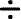Skill 16A Fact Families Write a division fact that belongs in the same fact family as the following multiplication fact. 1. 5 x 6 = 30 __________   =   _____
 Complete the fact family below to find the missing number. 2.                28___ = 4 7 x 4 = 28 284 = 7 4 x 7 = 28 ?